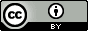## Saturday, October 4, 2014

### Tight binding DFT

Tight binding DFT (DFTB) is a semi-empirical method with speed and accuracy similar to NDDO-based semiempirical methods such as AM1, PM3, and PM6.  Currently there are three types of DFTB methods called DFT1, DFTB2, and DFTB3. DFTB1 and DFTB2 are sometimes called non-SCC DFTB (non-selfconsistent charge) and SCC-DFTB, respectively.  DFTB3 is generally considered the most accurate for molecules and there are several parameter sets for DFTB2 and DFTB3 for different elements.  Compared to PM6, DFTB has so far been parameterized for relatively few elements.

DFTB1
The closed shell DFTB1 energy is computed from the following equation
$$E^{\text{DFTB1}}=\sum_i^{N/2} \sum_\mu^K \sum_\nu^K 2 C_{\mu i} C_{\nu i} H^0_{\mu\nu}+\sum_A \sum_{B>A} E^{\text{rep}}_{AB}$$
where $C_{\mu i}$ is the molecular orbital coefficient for MO $i$ and basis function $\mu$.
$$H^0_{\mu\nu}= \begin{cases} \varepsilon^{\text{free atom}}_{\mu\mu} & \text{if } \mu=\nu \\ 0 & \text{if } A=B, \mu\ne\nu\\ \langle \chi_\mu | \hat{T}+V_{\text{eff}}[\rho_0^A+\rho_0^B] | \chi_\nu \rangle & \text{if } A\ne B \end{cases}$$
Here,  $\varepsilon^{\text{free atom}}_{\mu\mu}$ is an orbital energy of a free atom, $\chi$ is a valence Slater-type orbital (STO) or numerical orbital, $\hat{T}$ is the electronic kinetic energy operator, $V_{\text{eff}}$ is the Kohn-Sham potential (electron-nuclear attraction, electron-electron repulsion, and exchange correlation), and $\rho_0^A$ is the electron density of neutral atom $A$.

DFT calculations on free atoms using some functional yield $\left\{\varepsilon^{\text{free atom}}_{\mu\mu} \right\}$, $\left\{\chi\right\}$, and $\rho_0$, which are then used to compute $H^0_{\mu\nu}$ for A-B atom pairs at various separations $R_{AB}$ and stored. When performing DFTB calculations $H^0_{\mu\nu}$ is simply computed for each atom pair A-B by interpolation using this precomputed data set.

Similarly, the overlap matrix $\left\{ \langle \chi_\mu | \chi_\nu \rangle \right\}$ need to orthonormalize the MOs are computed for various distances and stored for future use.

$E^{\text{rep}}_{AB}$ is an empirical repulsive pairwise atom-atom potential with parameters adjusted to minimize the difference in atomization energies, geometries, and vibrational frequencies computed using DFTB and DFT or electronic structure calculations for set of molecules.

So, a DFTB1 calculation is performed by constructing $\mathbf{H}^0$, diagonalizing it to yield $\mathbf{C}$, and then computing $E^{\text{DFTB1}}$.

DFTB2
$$E^{\text{DFTB2}}=E^{\text{DFTB1}}+\sum_A \sum_{B>A} \gamma_{AB}(R_{AB})\Delta q_A\Delta q_B$$
where $\Delta q_A$ is the Mulliken charge on atom $A$ and $\gamma_{AB}$ is a function of $R_{AB}$ that tends to $1/R_{AB}$ at long distances.

The Mulliken charges depend on $\mathbf{C}$ so a selfconsistent calculation is required:
1. Compute DFTB1 MO coefficients, $\mathbf{C}$
2. Use $\mathbf{C}$ to compute $\left\{ \Delta q \right\}$
3. Construct and diagonalize $H_{\mu \nu}$ to get new MO coefficients, $\mathbf{C}$
$$H_{\mu \nu}=H_{\mu \nu}^0 + \frac{1}{2} S_{\mu\nu} \sum_C (\gamma_{AC}+\gamma_{BC})\Delta q_C, \mu \in A, \nu \in B$$
4. Repeat steps 2 and 3 until selfconsistency.

DFTB3
$$E^{\text{DFTB3}}=E^{\text{DFTB2}}+\sum_A \sum_{B>A} \sum_{C>B>A} \Gamma_{AB}\Delta q_A^2\Delta q_B$$
$\Gamma_{AB}$ is computed using interpolation using precomputed data. A SCF calculation is required.

Parameter sets and availability
DFTB is available in a variety of software packages.  I don't believe DFTB3 is currently in Gaussian and DFTB is also available in CHARMM and CP2K.  DFTB will soon be available in GAMESS.

Note that each user/lab must download the parameter file separately here.  There are several parameter sets.  The most popular sets for molecules are the MIO (materials and biological systems) for DFTB2 and 3OB (DFT3 organic and biological applications).

Dispersion and hydrogen bond corrections
Just like DFT and PM6, the DFTB can be corrected for dispersion and hydrogen bond effect.